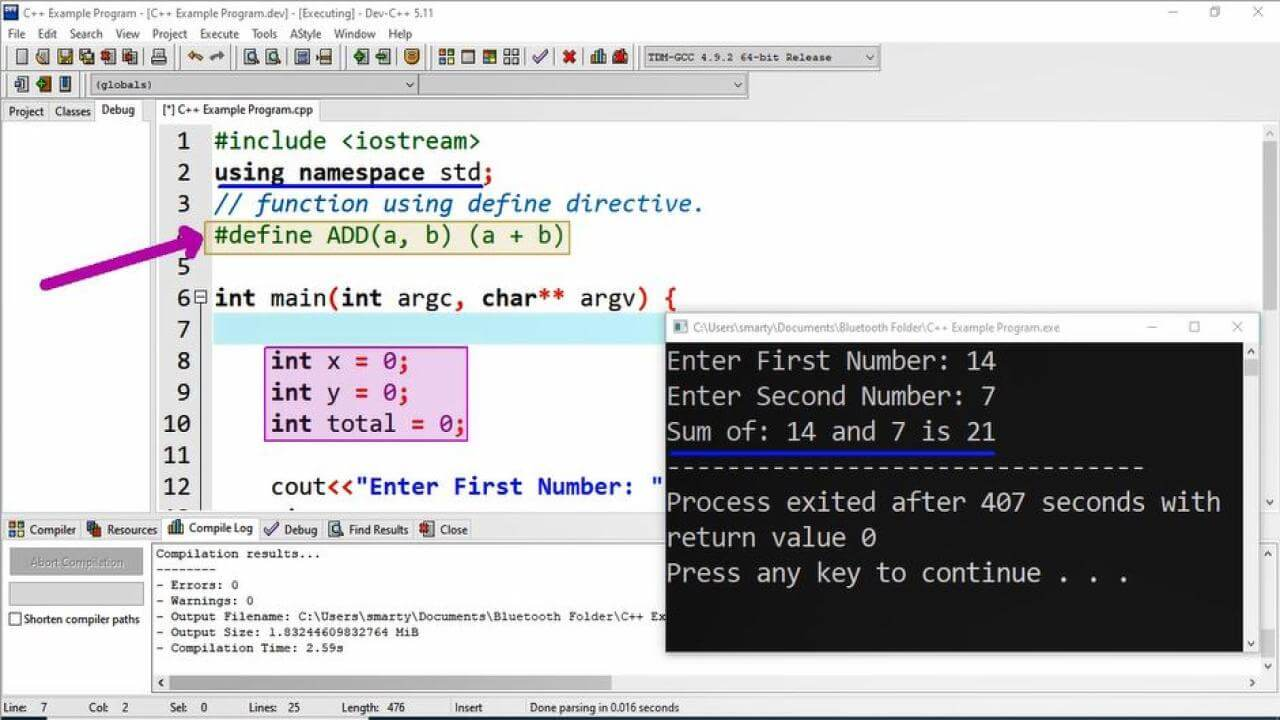# CPP program to Add Function Of Two Numbers Using Define Directive

## Source Code

#include <iostream>

using namespace std;

// function using define directive.
#define ADD(a, b) (a + b)

int main(int argc, char** argv) {

int x = 0;
int y = 0;
int total = 0;

cout<<"Enter First Number: ";
cin>>x;
cout<<"Enter Second Number: ";
cin>>y;

// function call

// printing result using cout statement.
cout<<"Sum of: "<<x<<" and "<<y<<" is "<<total;

return 0;
}

## Output## Working

In this program example we write a function that will return sum of two values using define directive.The most common way to write function is with the help of function declaration, function header and function body.

We have declared here three variables of int data type. Two variables named as x and y are used to take input from the user and the third variable is total. This total variable is used to store the return value from the function. The value returned from the function is stored in total variable using assignment oprator.

In last statement of this program example sum of input values are displayed on the screen using cout statement. Similarly we can define other functions using define directive for other mathematical operations.

 Sr. Operation Method Name Defination 1 Subtraction SUB #define SUB(a, b) (a - b) 2 Multiplication MUL #define MUL(a, b) (a * b) 3 Division DIV #define DIV(a, b) (a / b) 4 Modulus MOD #define SUB(a, b) (a % b)

In above list we can also use identifier of function name differently, But name will be valid identifier according to the C++ naming rules.